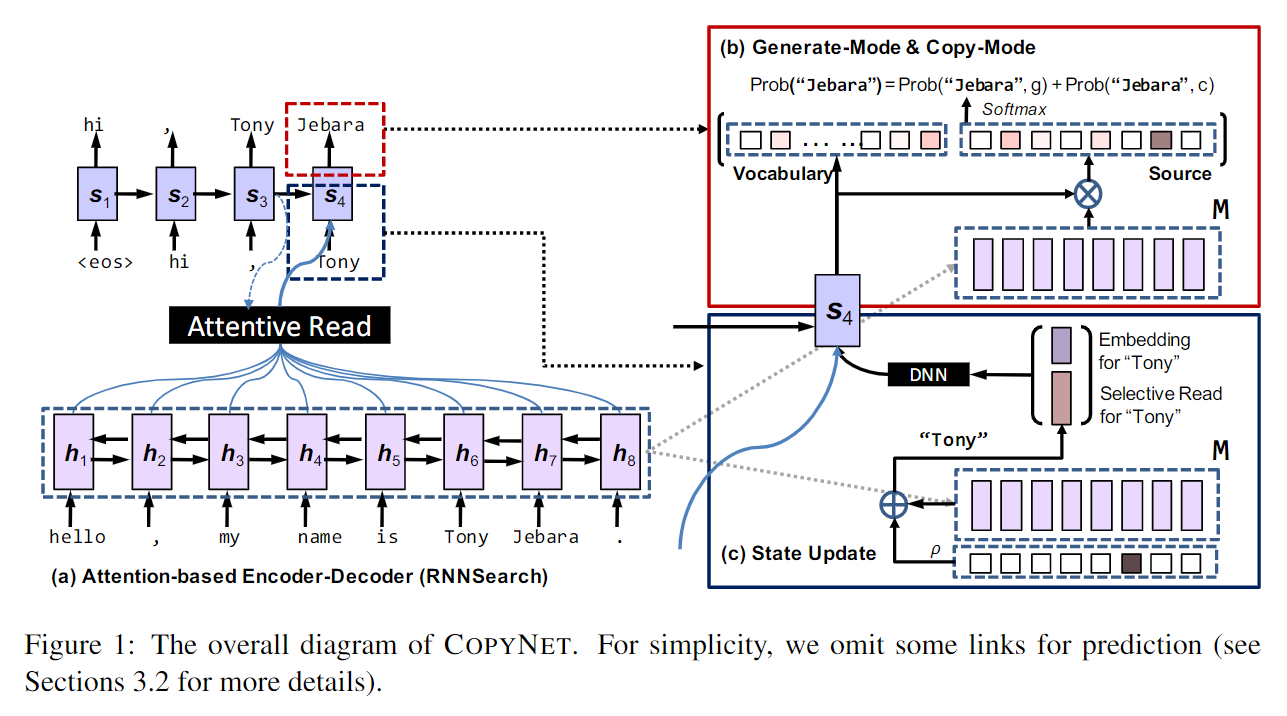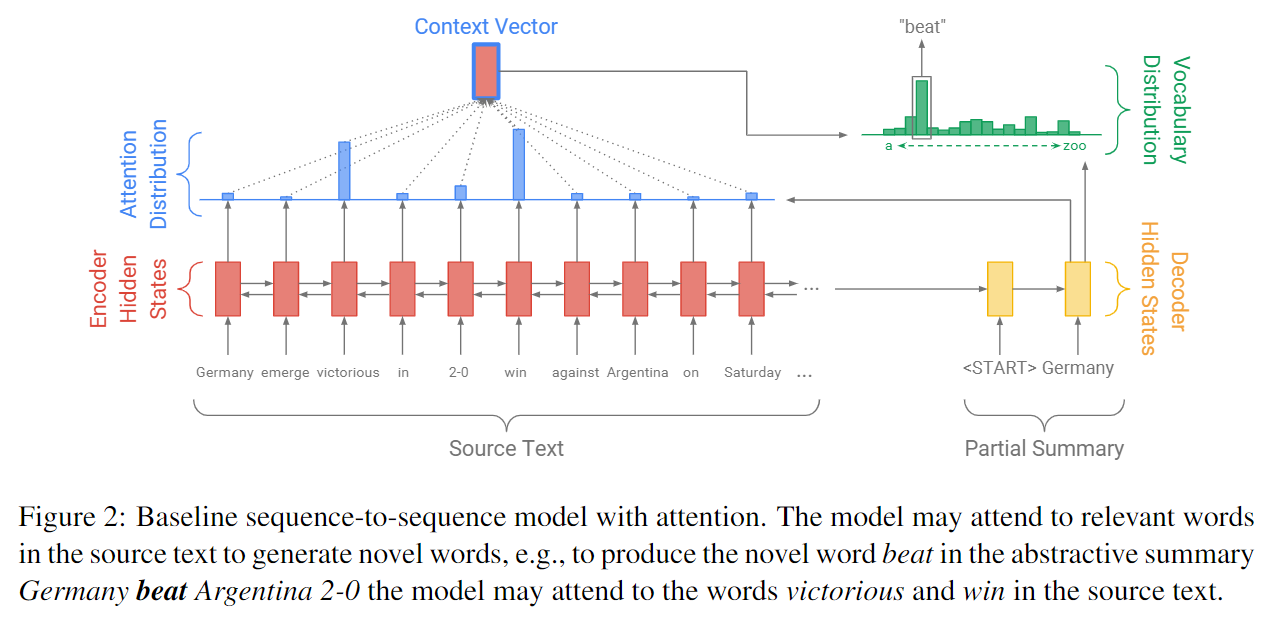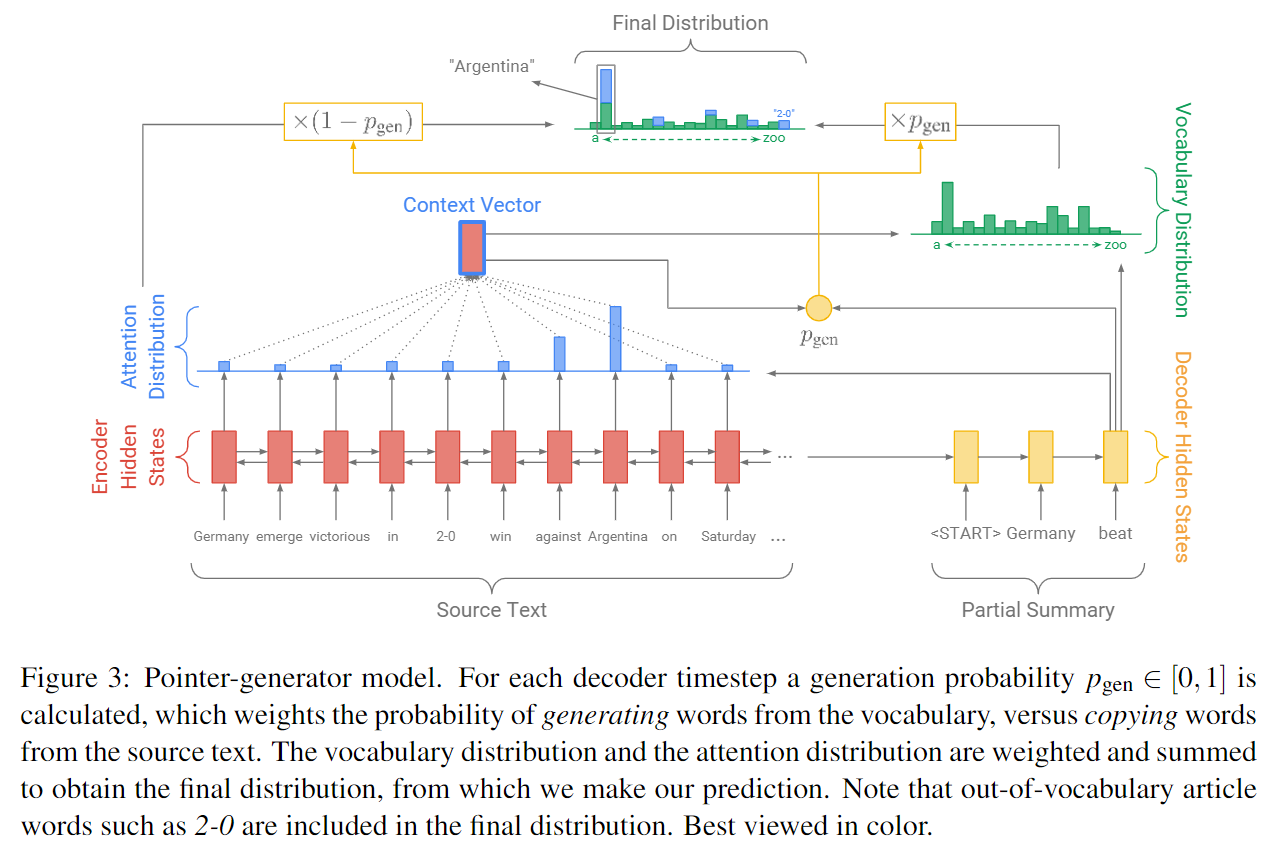# copy(pointer) making the seq2seq with attention better

2019/11/19

## Incorporating Copying Mechanism in Sequence-to-Sequence Learning

### RNN Encoder-Decoder with attention

Encoder端，输入序列$X=\left[ x_1,\cdots ,x_{T_s} \right]$被编码成相应的隐状态(向量)序列$h_1,\cdots ,h_{T_s}$，其中$h_t=f\left( x_t,h_{t-1} \right)$$h_0$为随机初始化的编码器端的隐状态。

Decoder端，首先根据上一时刻解码器的输出$y_{t-1}$、隐状态$s_{t-1}$以及当前时刻由编码器生成的隐状态序列对应的context向量$c_t$，更新当前时刻解码器的隐状态$s_t$。即

### copyNet• Prediction： copyNet在预测某个token时是基于生成模式和copy模式两种混合概率进行建模的，即
• State Update： 在RNN Encoder-Decoder with Bahdanau attention中，当前时刻解码器的状态$s_t$根据上一时刻解码器的输出$y_{t-1}$、隐状态$s_{t-1}$以及当前时刻由编码器生成的隐状态序列对应的context向量$c_t$进行更新；而在copyNet中，将$y_{t-1}$替换成$y_{t-1}$的embedding$e\left( y_{t-1} \right)$$y_{t-1}$在输入序列中对应的隐状态向量(若decoder在上一时刻的输出$y_{t-1}$不在encoder的输入序列$X=\left[ x_1,\cdots ,x_{T_s} \right]$中，则对应的隐状态向量为零向量)$\zeta \left( y_{t-1} \right)$的拼接$\left[ e\left( y_{t-1} \right) ;\zeta \left( y_{t-1} \right) \right] ^T$(此项体现了copy的思想)，其它项不变，即
• Reading M： 在解码端更新当前时刻的隐状态时，除了像上述attention机制，每次动态地将encoder端生成的所有隐状态向量序列($M$)表示成(加权求和，attentive read)该时刻对应的context向量外，还会检查解码器上一时刻的输出$y_{t-1}$在输入序列$X$中的位置(同一个词可能会出现多次)，然后在$M$取出(selective read)相应位置对应的隐状态向量$\zeta \left( y_{t-1} \right)$，不在的话，对应隐状态为零向量。 $M$的两种读取方式(attentive read与selective read，本质上都是基于注意力进行相关运算的)使得copyNet可以在Generate-Mode与Copy-Mode间进行切换，甚至决定何时开始或者结束copy。Attentive Read就是在编码器端的attention mechanism，Selective Read就是 $\zeta \left( y_{t-1} \right)$的计算过程。如果$y_{t-1}$在输入序列$X$中,那么copyNet接下去的输出就很可能偏向Copy-Mode。

## Get To The Point: Summarization with Pointer-Generator Networks

### Sequence-to-sequence attentional model### Pointer-generator network